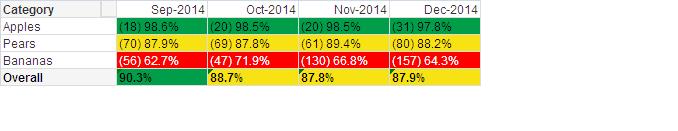Announcements
You can succeed best and quickest by helping others to succeed. Join the conversation.
cancel
Showing results for
Did you mean:Not applicable

## Show all months in pivot chart regardless of selections

Hello all

Please can you advise me (a novice!) on how I can keep all the months all visible in my pivot chart below, but still make other selections dependent on selections in other sheets (as is the default)?

On the other sheets you can only select one month at a time (which is what I want) - but this chart below switches between a pivot chart and a line chart to show the differences over time, so I always want all the months showing....Thanks

Chloe

1 Solution

Accepted SolutionsMVP

sum(NonCount)

try

sum({<Month = >}NonCount)

at every place

5 RepliesNot applicable
Author

sorry - screen shot didn't attach...MVP

Consider that you have

below dimensions

Item

Year

and below expression

SUM(Sales)

....

If you want TotalSales for all Years even though particular year is selected, you need to bypass Year using set analysis in expression... like below

SUM({<Year=>}Sales)

If you have MonthYear field, you can use the same instead of Year as shown in above example.

To bypass multiple field.. use can use as

SUM({<Field1 =, Field2 =, Field3= >}Sales)Not applicable
Author

Thanks Manish, but I am having trouble putting this into the expression i have:

IF(Dimensionality() = 0,
IF(
'('&sum(NonCount)&')' & ' ' &
num(sum(CompCount)/(Sum(NonCount)+Sum(CompCount)),'#,##0.0%') = '(0) ', 'N/A',
num(sum(CompCount)/(Sum(NonCount)+Sum(CompCount)),'#,##0.0%'))
,

IF(
'('&sum(NonCount)&')' & ' ' &
num(sum(CompCount)/(Sum(NonCount)+Sum(CompCount)),'#,##0.0%') = '(0) ', 'N/A', '('&sum(NonCount)&')' & ' ' &
num(sum(CompCount)/(Sum(NonCount)+Sum(CompCount)),'#,##0.0%')))

my dimensions are MONTH and Compliance Category

Are you able to advise on how i insert the {MONTH=>} into the expression below? when I do it brings out incorrect data for the selection that is made?

Thanks for your help so far

ChloeMVP

sum(NonCount)

try

sum({<Month = >}NonCount)

at every placeNot applicable
Author

Fab - thank you so much!Community Browser